# Sarngadeva’s Taana Prastaar – Part II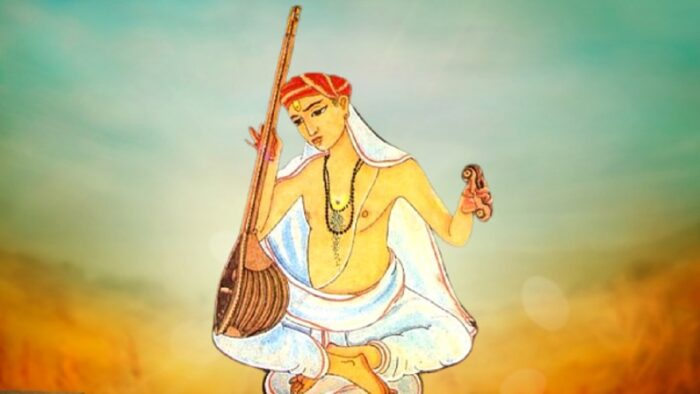This is in continuation of my earlier article – Sarngadeva’s Taana Prastaar, Part 1.

Given a taana what will be its row number in the taana prastaar and given a row number what will be the arrangement of the svaaras in that taana? Sarngadeva had given the Uddishta (उद्दिष्ट) and Nashtam (नष्टम्) pratyayas respectively to answer these questions. Before presenting the sutras for these pratyayas, let us understand the properties of factorials on which these pratyayas are based.

We have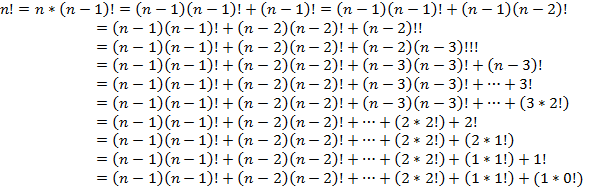Thus, we have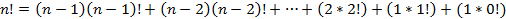One more interesting property of factorials:

Any integer m, where 1 <= m <= n!, can be uniquely represented in the form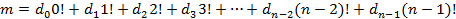Where,are integers where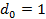and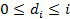for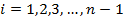Let us understand this with examples: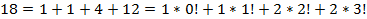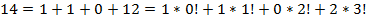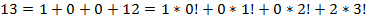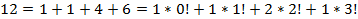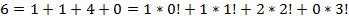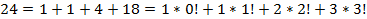Recall that in part 1 of this article I had mentioned that the 4 svara taana prastaar will have four 3 svara prastaars embedded within it. Each of these 3 svara prastaars will in turn have three 2 svara prastaars embedded within them.

Now let us use this wisdom to find the row number in the prastaar for a given taana.

Let us find the row number for the taana M R S G.

The given taana is from a 4 svara taana prastaar consisting of the svaras S R G M

Let the row number be represented by n = 0.

• The last svara in the taana is G. G is second highest amongst the svaras in the prastaar. Hence, n = 1 * 3! = 6.
• The 2nd svara from the right is S. S is the lowest amongst the remaining three svaras S R M. Hence, n = 6 + 2 * 2! = 6 + 4 = 10.
• The 3rd svara from the right is R is the lowest of the two remaining svaras R M. Hence, n = 10 + 1 * 1! = 10 + 1 =11
• Lastly, n = 11 + 1 * 0! = 11 + 1 = 12

We have, n = 1 * 3! + 2 * 2! + 1 * 1! + 1 * 0! = 6 + 4 + 1 + 1 = 12

Hence the row number of the taana M R S G = 12.

Verify the answer in the 4 svara taana prastaar given in Table 1.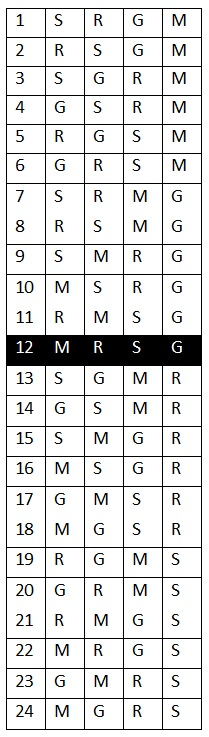Table 1

Now let us find the row number for the taana M P G R S.

The given taana is from a 5 svara taana prastaar consisting of the svaras S R G M P

Let the row number be represented by n = 0.

• The last svara in the taana is S. S is the lowest amongst the 5 svaras in the prastaar. Hence, n = 4 * 4! = 96.
• The 2nd svara from the right is R. R is the lowest amongst the remaining 4 svaras R G M P. Hence, n = 96 + 3 * 3! = 96 + 18 = 114.
• The 3rd svara from the right is G, which is the lowest of the 3 remaining svaras G M P. Hence, n = 114 + 2 * 2! = 114 + 4 = 118.
• The 4th svara from the right is P, which is the highest of the 2 remaining svaras M P. Hence, n = 118 + 0 * 1! = 118 + 0 = 118.
• Lastly, n = 118 + 1 * 0! = 118 + 1 = 119

We have, n = 4 * 4! + 3 * 3! + 2 * 2! + 0 * 1! + 1 * 0! = 96 + 18 + 4 + 0 + 1 = 119

Hence the row number of the taana M P G R S = 119.

Sarngadeva had created a table of factorials and their multiples called the Khandmeru (खण्डमेरु) to be used for his Uddishta (उद्दिष्ट) and Nashtam (नष्टम्) pratyayas. Sarngadeva’s sutra for the construction of Khandmeru:

सप्ताद्धेकान्तकोष्ठानामधोऽधः सप्त पङ्क्तयः॥

तास्वाद्धायामाद्धकोष्ठे लिखेदेकं परेषु खम्।

वेद्धतानस्वरमितान् न्यसेत् तेष्वेव लोष्टकान्॥

प्राक्पङ्क्त्यन्त्याङ्कसंयोगमूर्ध्वाधः स्थितपङ्क्तिषु।

शून्यादधो लिखेदेकं तं चाधोऽधः स्वकोष्ठकन्॥

कोष्ठसङ्ख्यागुणं न्यस्येत् खण्डमेरुरयं मतः। (सङ्गीतरत्नाकरः १.४.६३-६६)

Construct a 7 x 7 table as shown in Table 2. Place a 1 followed by six 0s in the first row. Place the factorials of 1 to 6 in the 2nd row starting from the 2nd column. Place twice, thrice etc., of the factorials in the succeeding rows, starting from a succeeding column at each stage.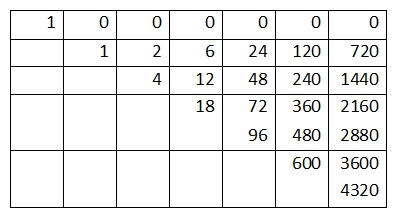Table 2

Let me now present the Sarngadeva’s sutra for his Uddishta pratyaya:

स्वरान् मूलक्रमस्यान्त्यात् पूर्वं यावतिथः स्वरः॥

उद्दिष्टान्त्यस्तावतिथे कोष्ठेऽधौ लोष्टकं क्षिपेत्।

लोष्टचालनमन्त्यात् स्यात्त्यक्त्वा लब्धं क्रमो भवेत्॥

लोष्टाक्रान्ताङ्कसंयोगादुद्दिष्टस्य मितिर्भवेत्।   – सङ्गीतरत्नाकरः  १.४.६६-६८

Given a taana (of n svaras), note the rank of the last svara in the reverse of natural order among the given svaras. Mark the corresponding entry in the the n-th column of the Khandmeru. Then note the rank of the next (second last) svara (in the reverse of natural order) among the remaining svaras. Mark the corresponding entry in the next or the (n – 1)th column of the Khandmeru. Then note the rank of the next (third last) svara (in the reverse of natural order) among the remaining svaras. Mark the corresponding entry in the next or the (n – 2)th column of the Khandmeru. This procedure goes on till you have processed all the svaras in the taana. The uddishta or the row-number of the given taana will be the sum of all the marked entries.

Let us find the row number for the taana M R S G with the help of Sarngadeva’s uddishta pratyaya.

The last svara of the given taana is G. It’s rank amongst all the svaras in the taana is 2. Hence mark the corresponding entry in the 2nd row (since the rank is 2) of the 4th column (since it is a 4 svara taana) of the Khandmeru. Refer Table 3.

The second last svara of the given taana is S. It’s rank amongst the remaining svaras is 3. Hence mark the corresponding entry in the 3rd row (since the rank is 3) of the 3rd column.

The third last svara of the given taana is R. It’s rank amongst the remaining svaras is 2. Hence mark the corresponding entry in the 2nd row of the 2nd column.

The first and the remaining svara is M. It’s rank will obviously be 1. Mark the 1st row of the 1st column, which is 1 in value.

To get the row number, add up the values of all the marked entries.

Hence, n = 6 + 4 + 1 + 1 = 12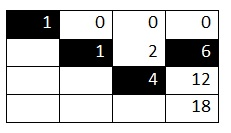Table 3

In the next and concluding part we will discuss Sarngadeva’s Nashta pratyaya for the Taana prastaar.

Disclaimer: The opinions expressed in this article belong to the author. Indic Today is neither responsible nor liable for the accuracy, completeness, suitability, or validity of any information in the article.